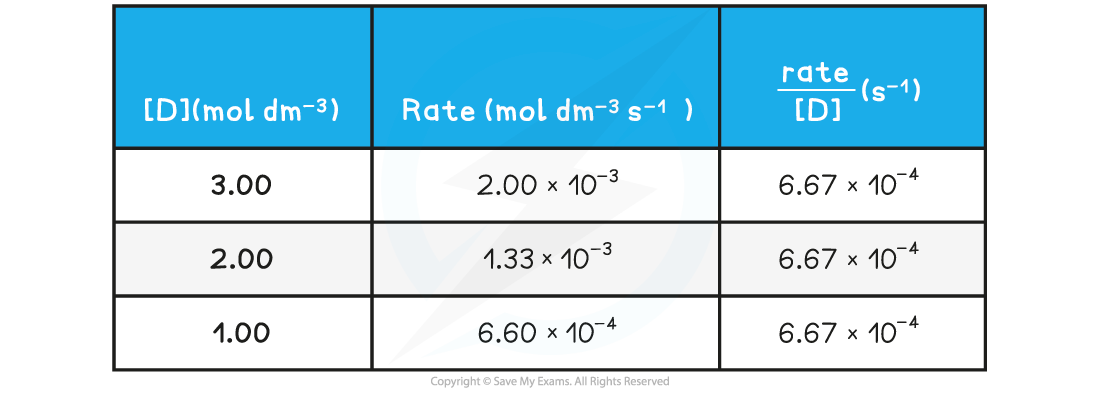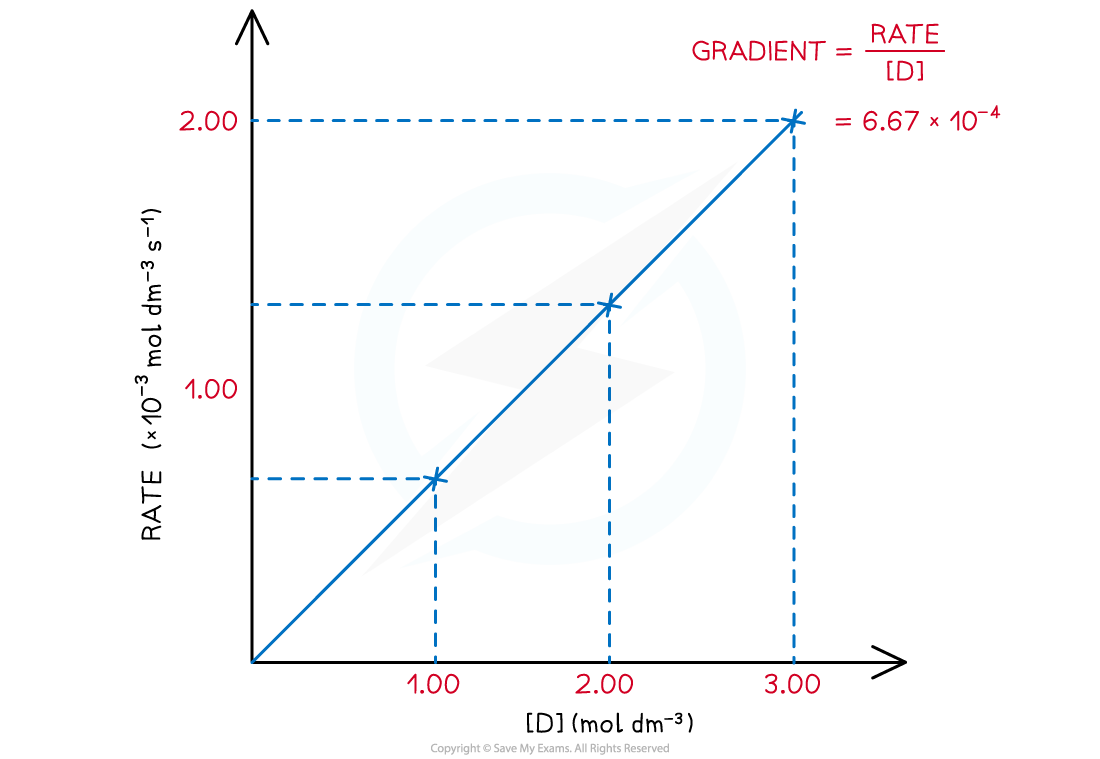# AQA A Level Chemistry复习笔记5.2.1 Rate Equations

### Rate Equations

• The rate of reaction refers to the change in the amount or concentration of a reactant OR product per unit time
• It can be found by:
• Measuring the decrease in the concentration of a reactant over time
• Measuring the increase in the concentration of a product over time
• The units for rate of reaction are mol dm-3 s-1

#### Rate equation

• The following general reaction will be used as an example to study the rate of reaction

D (aq) → E (aq) + F (g)

• The rate of reaction at different concentrations of D is measured and tabulated

Rate of reactions table• A directly proportional relationship between the rate of the reaction and concentration of D is observed when a graph is plottedRate of reaction over various concentrations of D

• Rate equations can only be determined experimentally and cannot be found from the stoichiometric equations

Rate of reaction = k [A]m [B]n

[A] and [B] = concentrations of reactants

m and n = orders of the reaction

• All of the reactant concentrations will have an order of 0, 1 or 2, depending on the effect that they have on the rate of the reaction
• The products are never involved in the rate equation, as they have no effect on the rate of the reaction
• For the above reaction, the rate equation would beRate = k [D]
• Let's take a real life example:

2NO (g) + 2H2 (g) → N2 (g) + 2H2O (g)

• The rate equation for the formation of nitrogen gas (N2) from nitrogen oxide (NO) and hydrogen (H2) is:

rate = k [NO]2 [H2]

• Notice that the [H2] does not have an order of 2
• This is because the order must be determined experimentally, not from the equation

• The orders of the reaction will be calculated from a table of experimental data, or from a graph
• The rate equation for the reaction above shows that:
• When changing the concentration of NO to determine how it affects the rate, while keeping [H2] constant
• The change in rate is proportional to the square of [NO]

Rate = k1 [NO]2

• And, when changing the [H2] to determine how it affects the rate while keeping [NO] constant
• The change in rate is proportional to [H2]

Rate = k2 [H2]

• Combining the two equations gives the overall rate equation (where k = k1 + k2)

Rate = k [NO]2 [H2]

• For a catalyst to appear in the rate equation:
• It must have a measurable and quantifiable effect on the rate of reaction
• The catalyst must be homogeneous
• If a chemical appears in a rate equation but is not one of the reactants, then it is a catalyst

#### Order of reaction

• The order of a reactant shows how the concentration of a reactant affects the rate of reaction
• It is the power to which the concentration of that reactant is raised in the rate equation
• The order can be 0, 1 or 2
• When the order of reaction of a reactant is 0, this means that it has no effect on the rate of the reaction and therefore is not included in the rate equation at all
• When the order of reaction of a reactant is 1, the rate is directly proportional to the concentration of that reactant
• When the order of reaction of a reactant is 2, the rate is directly proportional to the square of the concentration of that reactant

• The overall order of reaction is the sum of the powers of the reactants in a rate equation
• For example, in the following rate equation, the reaction is:

Rate = k [NO]2 [H2]

• Second-order with respect to NO
• First-order with respect to H2
• Third-order overall (2 + 1)

#### Half-life

• The half-life (t1/2) is the time taken for the concentration of a limiting reactant to become half of its initial value